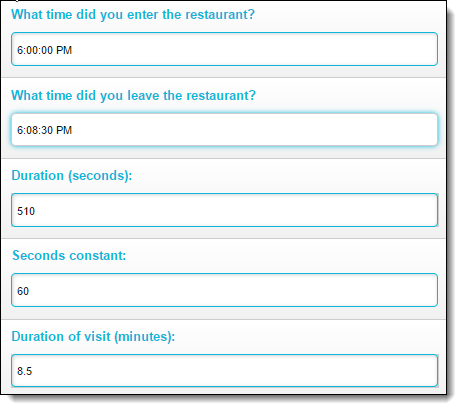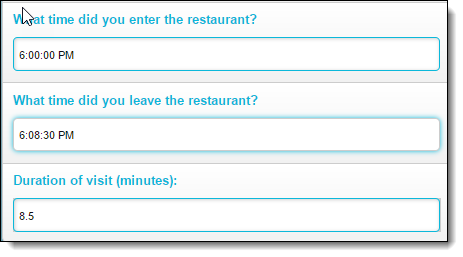## Announcing calculation question type!

We've just added a new question type: Calculation questions, which can:

• Add, subtract, multiply, divide or average the responses to two or more Number questions
• Calculate the duration between two Timestamp questions

Using a Calculation question is easy!  Just follow these steps:
1. Select Calculation in the question type dropdown
2. Select the operation that you would like the calculation question to perform
3. Select the Number or Timestamp questions on which to perform the calculationIf you're subtracting, dividing or calculating duration, the order of the operand questions matters!  It's the first question minus the second question, or the first question divided by the second question, or the second timestamp minus the first timestamp.

Hey, wanna see something cool?

Just as with any other type of question, you can combine Calculation questions with Presto Logic to get the effect you want.  For example, suppose you have a duration question, because I want to calculate the time that the shopper spent in the restaurant.  That's great...but the result of the calculation is shown in seconds.  Not very helpful -- you want to see the duration in minutes!To do this, add two more questions: first, a Number question for the number of seconds in a minute...and because you don't really want the shopper to tell me how many seconds are in a minute, use a Presto Logic Always Trigger rule to set the value of this question to 60 in all cases:Next, add another Calculation question that divides the result of the previous Calculation question (duration) by the Number question whose value is set to 60:...and now you can see the duration in minutes!Now let's add one last touch: the shopper doesn't need to see the duration in seconds or the seconds constant, so let's hide them in Presto Logic:The final result: a nice clean-looking survey!Not sure how to use Calculation questions?  Contact our support department at support@prestomobilesurveys.com - we'd be glad to help!